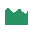This site uses cookies. By continuing to browse the ConceptDraw site you are agreeing to our Use of Site Cookies.

# Histogram Chart

What is a Histogram Chart? It is a chart which visually displays a distribution of the data. ConceptDraw DIAGRAM extended with Histograms solution from the Graphs and Charts area of ConceptDraw Solution Park is the best software for fast and simple drawing professional looking Histogram Chart.

## Make a Histogram

ConceptDraw DIAGRAM extended with Histograms solution from the Graphs and Charts area of ConceptDraw Solution Park lets you make a Histogram of any complexity in minutes.

## How To Draw a Histogram?

How to draw a Histogram? It's very fast and easy to draw any Histogram using the tools of ConceptDraw DIAGRAM software extended with Histograms solution from the Graphs and Charts area of ConceptDraw Solution Park.
HelpDesk

## How to Draw a Histogram

Histogram is a diagram used to visualize data through bars of variable heights. Making histogram can be helpful if you need to show data covering various periods (hours, days, weeks, etc). When the vertical column of the histogram refer to frequency it shows how many times any event happens. It is a Frequency histogram. A bar chart diagram is similar to a histogram, but in contrast to a bar chart a histogram represents the number how many times there have been certain data values. You can use a histogram to depict continual data flow such as temperature, time, etc. You can effortlessly draw histograms using the Histograms solution for CnceptDraw DIAGRAM. Making a histogram can by very useful to represent various statistical data.

## Histogram

Any histogram is known to be an accurate representation of the distribution of numerical data. Being an estimate of the probability distribution of a continuous variable, the histogram was first introduced by Karl Pearson as a kind of a bar graph. To make a histogram, you need to divide the range of values into a series of intervals and then to count how many of the values fall into each of the intervals.

##Histograms

How to make a Histogram? Making a Histogram is an incredibly easy process when it is done with ConceptDraw DIAGRAM. The Histograms Solution enhances ConceptDraw DIAGRAM functionality with extensive drawing tools, numerous samples, and examples; also a quick-start template and library of ready vector stencils for visualization the data and professional drawing Histograms.

##Basic Histograms

This solution extends the capabilities of ConceptDraw DIAGRAM (or later) with templates, samples and a library of vector stencils for drawing Histograms.

## How To Make a Histogram?

How to make a Histogram quick and easy? ConceptDraw DIAGRAM diagramming and vector drawing software extended with Histograms solution from the Graphs and Charts area will effectively help you in Histogram drawing.

## Making a Histogram

Being is an accurate representation of some distribution of numerical data, any histogram is one of the most commonly used drawings that can be also created with the help of the ConceptDraw DIAGRAM diagramming and drawing software. Having the needed solution from the Graphs and Charts area of ConceptDraw STORE application, making a histogram can be a fun and entertaining process as all that has to be done is editing the already existing templates that were previously created by the team of the It specialists of CS Odessa.

## Seven Basic Tools of Quality - Histogram

A histogram is a chart which visually displays a distribution of numerical data.
ConceptDraw DIAGRAM diagramming and vector drawing software offers a powerful Seven Basic Tools of Quality solution from the Quality area of ConceptDraw Solution Park which contains a set of useful tools for easy drawing Histogram and other quality related diagrams of any complexity.